# Efficient Reconstruction of Metabolic Pathways by Bidirectional Chemical Search

## Abstract

One of the main challenges in systems biology is the establishment of the metabolome: a catalogue of the metabolites and biochemical reactions present in a specific organism. Current knowledge of biochemical pathways as stored in public databases such as KEGG, is based on carefully curated genomic evidence for the presence of specific metabolites and enzymes that activate particular biochemical reactions. In this paper, we present an efficient method to build a substantial portion of the artificial chemistry defined by the metabolites and biochemical reactions in a given metabolic pathway, which is based on bidirectional chemical search. Computational results on the pathways stored in KEGG reveal novel biochemical pathways.

## 1. Introduction

Metabolism can be regarded as a network of chemical reactions activated by enzymes and connected via their substrates and products, and a metabolic pathway can be regarded as a coordinated sequence of biochemical reactions (Deville et al., 2003). The definition of a metabolic pathway is not exact, and most pathways constitute indeed highly intertwined cyclic networks. In a cell, the substrates of a pathway are usually the products of another pathway, and there are junctions where pathways meet or cross (Karp and Mavrovouniotis, 1994).

The analysis of metabolic pathways is motivated by the rapidly increasing quantity of available information on metabolic pathways for different organisms. One of the most comprehensive sources of metabolic pathway data is the Roche Applied Science Biochemical Pathways chart (Michal, 1999). There are also several databases on metabolic pathways, such as aMAZE (Lemer et al., 2004), BRENDA (Schomburg et al., 2002), MetaCyc (Caspi et al., 2006), KEGG (Kanehisa and Goto, 2000), and WIT (Overbeek et al., 2000). These databases contain hundreds of metabolic pathways and thousands of biochemical reactions, and even the metabolic pathway for a small organism constitutes a large network. For instance, the proposed metabolic pathway for the bacterium E. coli consists of 436 compounds (substrates, products, and intermediate compounds) linked by 720 reactions (Edwards and Palsson, 2000).

An artificial chemistry (Dittrich et al., 2001), on the other hand, is a computational model of a chemical system that consists of a set of objects (molecules), a set of reaction rules (that allow for the production of new molecules from already existing molecules), and a definition of the dynamics of the system (that is, application conditions for the reaction rules), aimed at answering qualitative questions about the chemical system. Thus, artificial chemistries model real chemistries, in which molecules represent chemical compounds and reaction rules represent chemical reactions and, in particular, artificial chemistries model organic chemistries (Benkö et al., 2003a, 2003b, 2004).

The chemical description of molecules in an artificial chemistry can be made at different levels of resolution, from simple molecular descriptors to structural formulas. One of these representations are chemical graphs, with nodes corresponding to the atoms of the molecules and edges indicating the bonds between them. Chemists have used chemical graphs to distinguish isomers since the second half of the nineteenth century, and in first course organic chemistry classes, chemical reactions are explained in terms of constitutional formulas and a handful of reaction mechanisms, which are nothing but chemical graphs and rules to modify them by means of breaking, forming, and changing the type of bonds. This leads in a natural way to artificial chemistries based on labeled graphs as molecules and graph transformation rules as reactions. Several such artificial chemistries have been proposed so far: see, for instance, (Benkö et al., 2003a, 2003b, 2004; McCaskill and Niemann, 2001; Rosselló and Valiente, 2005a).

Artificial chemistries can also be used to model biochemical systems such as metabolic pathways, in which molecules represent metabolites and reaction rules represent biochemical reactions (Rosselló and Valiente, 2005b), and they allow for answering qualitative questions about metabolism. In this paper, we present an efficient method to build a substantial portion of the artificial chemistry defined by the metabolites and biochemical reactions in a given metabolic pathway. Our method is based on bidirectional chemical search, and its implementation uses chemical graphs to represent sets of molecules. We report also on the results of some experiments applying this method to pathways stored in KEGG, which reveal novel biochemical pathways.

## 2. Modeling biochemical reactions as chemical graph transformations

Following (Rosselló and Valiente, 2005a), by a chemical graph, we understand a complete labeled weighted graph (V,E, ℓ,μ), with (V, E) an undirected graph (without multiple edges or self-loops), a labeling mapping that labels every node vV with a chemical element (υ), and μ : E → ℕ an edge weight function. We shall denote the weight of the edge joining nodes υ and w by μ(υ, w); notice that μ(υ, w) = μ(w, υ) because the graph is undirected. A weight of 0 stands for a nonexisting bond, a weight of 1 for a single bond, a weight of 2 for a double bond, etc. The valence of a node in a chemical graph is the total weight of the edges incident to it.

To simplify the language, we shall call a multi-molecule to any set of molecules. Such a multi-molecule is described by the disjoint union of the chemical graphs representing the molecules and then adding weight 0 edges between atoms of different molecules. In this way, the molecules in the set are identified as maximal connected subgraphs with nonzero weight edges; see Fig. 1.

Given two chemical graphs G 1 = (V 1,E 1, 1,μ 1) and G 2 = (V 2,E 2, 2,μ 2), an atom mapping between them is a bijection M : V 1V 2 such that, for every υ 1V 1:

• $${\ell _1}\left( {{\upsilon _1}} \right) = {\ell _2}\left( {M\left( {{\upsilon _1}} \right)} \right)$$.

• $$\sum\nolimits_{{w_1} \in {V_1}} {{\mu _1}\left( {{\upsilon _1},{w_1}} \right) = } \sum\nolimits_{{w_1} \in {V_1}} {{\mu _2}\left( {M\left( {{\upsilon _1}} \right),M\left( {{w_1}} \right)} \right)}$$.

When there exists an atom mapping between two chemical graphs G 1 and G 2, these chemical graphs (and the multi-molecules they represent) are said to be compatible: this means that they have the same number of nodes for each possible pair (label, valence). Notice that there is no stereochemical information in this simplified representation, and thus stereoisomers are represented by the same chemical graph. There is no electrical charge information either, and anions and cations are also represented by the same chemical graph.

A chemical reaction graph is a structure R = (G 1,G 2,M), where G 1 = (V 1, E 1, 1, μ 1) and G 2 = (V 2,E 2, 2,μ 2) are compatible chemical graphs, called the substrate and the product chemical graphs, respectively, and M : V 1V 2 is an atom mapping between them.

The application of a chemical reaction graph to a given chemical graph, consists of breaking, forming, and changing bonds in a subgraph of the chemical graph which is isomorphic to the substrate of the chemical reaction graph. Reversible chemical reaction graphs can also be applied in the opposite direction, by breaking, forming, and changing bonds in a subgraph of the chemical graph which is isomorphic to the product of the chemical reaction graph.

The size of an atom mapping M between two chemical graphs G 1 = (V 1,E 1, 1,μ 1) and G 2 = (V 2,E 2, 2,μ 2) is given by

$${\rm{size}}\left( M \right) = \sum\limits_{\left( {\upsilon ,w} \right) \in {E_1}} {\left| {{\mu _2}\left( {M\left( \upsilon\right),M\left( w \right)} \right) - {\mu _1}\left( {\upsilon ,w} \right)} \right|}$$

.

Given two compatible chemical graphs G 1 = (V 1,E 1, 1,μ 1) and G 2 = (V 2,E 2, 2 μ 2), an optimal atom mapping between them is an atom mapping of minimal size, which always exists (but it needs not be unique). An optimal atom mapping models the classical principle of minimum structure change, by which a chemical reaction normally occurs through the redistribution of the minimum number of valence electrons, that is, the formation and breaking of the least number of covalent bonds (Temkin et al., 1996).

The size of a chemical reaction graph R = (G 1,G 2,M) is simply the size of the corresponding atom mapping M.

## 3. Reconstructing metabolic pathways by bidirectional chemical search

Artificial chemistries (Dittrich et al., 2001) are computational models of chemical systems and, in particular, of biochemical systems such as metabolic pathways. An artificial chemistry consists of a set of molecules, a set of reaction rules that produce new molecules from already existing molecules, and the definition of the dynamics of the system, which specifies the application conditions of the rules, the preference in their application, etc. (Rosselló and Valiente, 2005b).

A metabolic pathway can be regarded as a coordinated sequence of biochemical reactions and is often described in symbolic terms, as a succession of transformations of one set of substrate molecules into another set of product molecules (Rosselló and Valiente, 2004). Substrate and product must be compatible chemical graphs for a pathway between them to exist (Rosselló and Valiente, 2004, 2005a, 2005b).

Metabolic pathways are often represented as directed hypergraphs, with substrate and product molecules as nodes and biochemical reactions as hyperarcs. Since a chemical graph can represent the disjoint union of a set of molecules, though, the equivalent representation of artificial chemistries and, in particular, metabolic pathways as directed graphs becomes more natural. An artificial chemistry defined by a set of chemical reaction graphs, is thus represented as a directed second-order graph with the chemical graphs that represent the sets of substrate and product molecules as vertices and applications of the chemical reaction graphs, including information on atom mapping, as arcs.

Unfortunately, the size of the artificial chemistry defined by a setM of chemical graphs and a set R of chemical reaction graphs is often exponential in the size of M and R, and thus artificial chemistries are known for very small instances only, involving a few dozens of molecules and biochemical reactions. Therefore, we consider in this paper the problem of obtaining a substantial portion of the artificial chemistry defined by a set of biochemical reactions while avoiding the complexity of reconstructing the whole artificial chemistry.

The constraints we impose on the reconstruction process are threefold:

1. (1)

The initial chemical graphs represent all sets of at most m metabolites among those involved in the set R of reactions, for some fixed, but arbitrary, m (in examples and applications in this paper we shall always take m = 2).

2. (2)

The reconstruction process is restricted to a fixed, but arbitrary, number k of derivation steps.

3. (3)

The initial and final sets of metabolites of every metabolic pathway belong to the set of initial chemical graphs.

While the first two constraints (on the size of the initial chemical graphs and the lengths of the metabolic pathways under inspection) are motivated by complexity considerations alone, the third constraint allows for directing the search of new metabolic pathways inside the artificial chemistry. That is, instead of building the artificial chemistry by applying the biochemical reactions in every possible way to each of the initial chemical graphs, we perform a bidirectional search by constructing forward metabolic pathways of length at most k starting in initial chemical graphs and backward metabolic pathways of length at most k ending in initial chemical graphs, and then gluing them to obtain all metabolic pathways of length at most 2k starting and ending in initial chemical graphs.

Given a set R of biochemical reactions and a number k of derivation steps, the detailed procedure for reconstructing all metabolic pathways of length up to 2k using the metabolites and reactions in R and starting and ending in multi-molecules of at most m components, is the following:

• First, we extract the set M of all chemical graphs representing sets of at most m any metabolites appearing in substrates and products of the reactions in R. We call the elements of M the initial chemical graphs.

• Next, we identify all compatibility classes in M (maximal subsets of compatible initial chemical graphs). Biochemical reactions transform chemical graphs into compatible chemical graphs and, therefore, the origin and the end of a metabolic pathway will be compatible sets of metabolites. Thus, since we restrict ourselves to metabolic pathways starting and ending in initial chemical graphs, we can restrict ourselves to search for metabolic pathways starting and ending in each compatibility class of initial chemical graphs.

• Then each compatibility class C in M is considered as a set of potential substrates C (0) F and a set of potential products C (0) R for the reactions in R.

• For every i = 1, …, k, the forward application of the reactions in R to the elements of C (i−1) F produces a set of multi-molecules C (i) F , while the reverse application of these reactions to the molecules in C (i−1) R produces a set of multi-molecules C (i) R .

• Any nonempty intersection of a set obtained by forward application and a set obtained by reverse application of reactions yields a new pathway between elements of C. To avoid repetitions, it is enough to check whether each C (i) F intersects C (i) R and C (i−1) R . More specifically:

• For i = 1, the forward application of the reactions in R to the molecules in C (0) F produces a set C (1) F of new molecules, and the reverse application of the reactions in R to the molecules in C (0) R produces a set C (1) R of new molecules.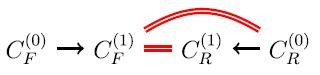Then

• Every member of C (1) F C (0) R yields a new pathway C (0) F C (1) F C (0) R of length 1.

• Every member of C (1) F C (1) R yields a new pathway C (0) F C (1) F C (1) R C (0) R of length 2.

• For i = 2, the forward application of the reactions in R to the molecules in C (1) F produces a set C (2) F of new molecules, and the reverse application of the reactions in R to the molecules in C (1) R produces a set C (2) R of new molecules.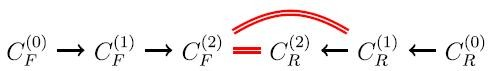Then

• Every member of C (2) F C (1) R yields a new pathway of length 3

$$C_F^{\left( 0 \right)} \to C_F^{\left( 1 \right)} \to C_F^{\left( 2 \right)} \cap C_R^{\left( 1 \right)} \to C_R^{\left( 0 \right)}$$

.

• Every member of C (2) F C (2) R yields a new pathway of length 4

$$C_F^{\left( 0 \right)} \to C_F^{\left( 1 \right)} \to C_F^{\left( 2 \right)} \cap C_R^{\left( 2 \right)} \to C_R^{\left( 1 \right)} \to C_R^{\left( 0 \right)}$$

.

• And, recursively, the forward application of the reactions in R to the molecules in I F = C (i−1) F produces a set C F = C (i) F of new molecules, and the reverse application of the reactions in R to the molecules in I R = C (i−1) R produces a set C R = C (i) R of new molecules.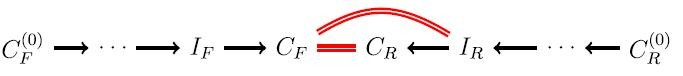Then

• Every member of C F I R yields a new pathway of length 2i − 1

$$C_F^{\left( 0 \right)} \to ... \to {I_F} \to {C_F} \cap {I_R} \to ... \to C_R^{\left( 0 \right)}$$

.

• Every member of C F C R yields a new pathway of length 2i

$$C_F^{\left( 0 \right)} \to ... \to {I_F} \to {C_F} \cap {C_R} \to {I_R} \to ... \to C_R^{\left( 0 \right)}$$

.

The following result shows that in this way we obtain all metabolic pathways of length at most 2k under constraints (1) and (3) above.

Lemma 1. For every i = 1, …, k, all metabolic pathways of length 2i−1 and 2i starting and ending in initial chemical graphs are obtained in the ith iterative step of the procedure explained above.

Proof: If

$${m_0} \to {m_1} \to ... \to {m_i} \to ... \to {m_{2i - 1}}$$

is a pathway with m 0 and m 2i−1 initial chemical graphs, then m j C (j) F for every j = 0, …, i and m 2i−1−l C (l) R for every l = 0, …, i − 1, and hence in particular, m i C (i) F C (i−1) R . Therefore, this path is obtained in the ith iterative step of the procedure explained above

On the other hand, if

$${m_0} \to {m_1} \to ... \to {m_i} \to ... \to {m_{2i}}$$

is a pathway with m 0 and m 2i initial chemical graphs, then m j C (j) F for every j = 0, …, i and m 2il C (l) R for every l = 0, …, i, and hence, in particular, m i C (i) F C (i) R . Therefore, this path is also obtained in the ith iterative step of that procedure.

Example 1. Let a, b, c, d, e, f be metabolites such that b, d, e, f are compatible with each other, a is compatible with b + b and c is compatible with b + b + b. Consider the toy artificial chemistry given by the following reactions (where only the first four reactions are reversible):

\eqalign{ & a + b \leftrightarrow c,a \leftrightarrow d + e,b + d \leftrightarrow b + e,b + b \leftrightarrow d + f,\cr & c \to e + b + b,d + d \to a,a + f \to b + e + e \cr}

Let us look for metabolic pathways starting and ending with metabolites and pairs of metabolites a, …, f globally compatible with b + b + b. Then the set M of all initial chemical graphs can be identified with the set of monomials of total weight at most 2 over the alphabet {a, b, c, d, e, f } and the class C of the initial chemical graphs compatible with bbb (we omit henceforth the + sign for simplicity) is

$$C = \left\{ {c,ab,ad,ae,af} \right\}$$

. So, we are looking for metabolic pathways starting and ending in elements of this set C. The intermediate multi-molecules of these pathways will belong to the set of all multimolecules formed by metabolites a, b, c, d, e, f compatible with bbb: these are the multimolecules in C plus any combination of three metabolites b, d, e, f.

Taking

$$C_F^{\left( 0 \right)} = C_R^{\left( 0 \right)} = C = \left\{ {a,ab,ad,ae,af} \right\}$$

, we obtain the following one step derivations:

Notice that some elements of C (1) F and C (1) R do no longer belong to M, as we warned

Then

$$C_F^{\left( 1 \right)} = \left\{ {c,ab,bbe,bde,bee,dde,dee,def} \right\}$$
$$C_R^{\left( 1 \right)} = \left\{ {c,ab,bdd,bde,ddd,dde,ddf,dee,def} \right\}$$

and hence

$$C_F^{\left( 1 \right)} \cap C_R^{\left( 0 \right)} = \left\{ {ab,c} \right\},C_F^{\left( 1 \right)} \cap C_R^{\left( 1 \right)} = \left\{ {ab,c,bde,dde,dee,def} \right\}$$

. From these intersections, we deduce that all metabolic pathways of lengths 1 and 2 starting and ending in C are

\eqalign{ & C_F^{\left( 1 \right)} \cap C_R^{\left( 0 \right)} = \left\{ {ab,c} \right\},C_F^{\left( 1 \right)} \cap C_R^{\left( 1 \right)} = \left\{ {ab,c,bde,dde,deee,def} \right\}\cr & c \to ab,ab \to c,c \to ab \to c,ab \to c \to ab,ab \to bde \to ab,\cr & ad \to dde \to ad,ad \to dde \to ae,ae \to dee \to ae,af \to def \to af \cr}

.

For k = 2, we obtain:

Then

$$C_F^{\left( 2 \right)} = \left\{ {c,ab,ad,ae,af,bbd,bbe,bdd,bde,bee,def} \right\}$$
$$C_R^{\left( 2 \right)} = \left\{ {c,ab,ad,ae,af,bbd,bbe,bdd,bde,bee} \right\}$$

and hence

$$C_F^{\left( 2 \right)} \cap C_R^{\left( 1 \right)} = \left\{ {c,ab,bdd,bde,def} \right\}$$

,

$$C_F^{\left( 2 \right)} \cap C_R^{\left( 2 \right)} = \left\{ {c,ab,ad,ae,af,bbd,bbe,bdd,bde,bee} \right\}$$

. From these intersections, we deduce that all metabolic pathways of lengths 3 and 4 starting and ending in C are

As it can be seen in the previous example, the raw application of the procedure explained above generates all metabolic pathways of length up to 2k starting and ending in sets of at most m metabolites used by the reactions in R, but most of these metabolic pathways will be redundant, for instance because they are cyclic, or because they do not contain any new multi-molecule that has not appeared in shorter metabolic pathways. Therefore, several reconstruction problems may be addressed in this context. In this work, we consider only three of them:

1. (a)

to produce all metabolic pathways of length up to 2k

2. (b)

to produce all shortest metabolic pathways of length up to 2k

3. (c)

to produce all minimal acyclic metabolic pathways of length up to 2k in all cases under restrictions (1) to (3) made explicit above.

Here, by a shortest metabolic pathway between metabolite sets I and F, we understand a metabolic pathway from I to F of shortest length among all metabolic pathways from I to F, and by a minimal acyclic metabolic pathway we understand a metabolic pathway that contain no directed cycles and no other, shorter metabolic pathways with intermediates in I or F. For instance, the shortest path derivation

$$ab \to c \to bbe \to def \to af$$

in Example 1 is acyclic but not minimal, because it contains the derivation cbbedefaf, while the minimal acyclic derivation

$$c \to bbe \to bbd \to ddf \to af$$

is not shortest, because there is a shorter derivation cbbedefaf from c to af .

We give our reconstruction algorithms in full pseudocode next. Algorithm 1 one formalizes the procedure explained above.

The first three lines of this algorithm produce the different compatibility classes of initial chemical graphs. Then for each compatibility class C and for each i = 1, …, k:

• It receives the sets I F = C (i−1) F and I R = C (i−1) R of the results of all direct and reverse applications, respectively, of i − 1 consecutive rules in R to multi-molecules in C (when i = 1, C (0) F = C and C (0) R = C) and it produces the sets N F = C (i) F and N R = C (i) R of the results of all direct and reverse applications, respectively, of rules in R to multimolecules in I F and I R , respectively. That is, the sets of the results of all direct and reverse applications, respectively, of i consecutive rules in R to multi-molecules in C.

• The lines starting with output call a procedure that outputs the list of all metabolic pathways of lengths 2i − 1 and 2i obtained so far. When i = 1:

• the first output line gives all length 1 pathways mm (1) f , with mC,

• the second output line gives all length 2 pathways mm (1) r m′ with m,m′ ∈ C.

And when i > 1:

Algorithm 1. Given a set R of biochemical reactions and a number k of derivation steps, obtain the set of all metabolic pathways of length up to 2k using the metabolites and reactions in R starting and ending in sets of at most m metabolites among those involved in the reactions in R.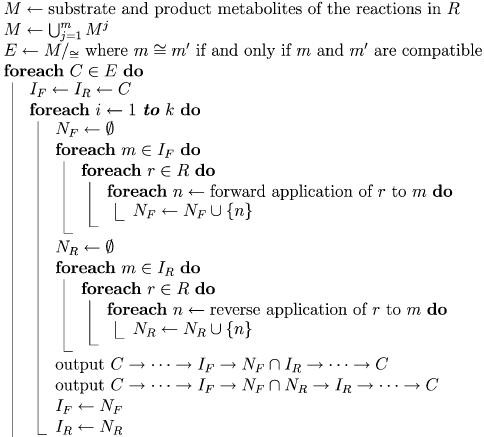• Thefirst output line gives all length 2i − 1 pathways

$$m \to m_f^{\left( 1 \right)} \to ... \to m_f^{\left( {i - 1} \right)} \to m_f^{\left( i \right)} = m_r^{\left( {i - 1} \right)} \to m_r^{\left( {i - 2} \right)} \to ... \to m_r^{\left( 1 \right)} \to m'$$

with m,m′ ∈ C.

• The second output line gives all length 2i pathways

$$m \to m_f^{\left( 1 \right)} \to ... \to m_f^{\left( {i - 1} \right)} \to m_f^{\left( i \right)} = m_r^{\left( i \right)} \to m_r^{\left( {i - 1} \right)} \to ... \to m_r^{\left( 1 \right)} \to m'$$

with m,m′ ∈ C.

Algorithm 2 produces a metabolic network (X, Y) containing all metabolic pathways up to a given length, where the vertex set X contains the initial and final metabolite sets together with all those new metabolite sets produced by the forward and reverse application of the given biochemical reactions, and the arc set Y consists of all direct derivations thus obtained.

Now, upon the metabolic network (X, Y ) obtained with the previous algorithm, the set of all shortest metabolic pathways of length up to 2k, using the metabolites and reactions in R starting and ending in sets of at most m metabolites among those involved in the reactions in R, can be obtained by using an all-pairs shortest path algorithm (Dijkstra, 1959; Floyd, 1962; Johnson, 1977; Takaoka, 1998) upon each element of C as source vertex and each element of C as target vertex in turn.

Algorithm 2. Given a set R of biochemical reactions and a number k of derivation steps, obtain the metabolic network (X, Y) containing all metabolic pathways of length up to 2k, using the metabolites and reactions in R starting and ending in sets of at most m metabolites among those involved in the reactions in R.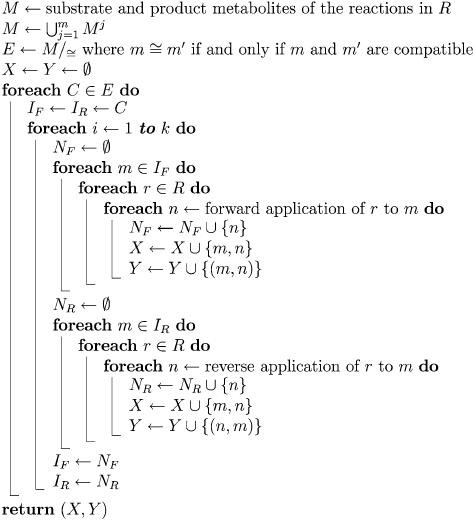Example 2. The toy artificial chemistry of Example 1, obtained from the class C = {c, ab, ad, ae, af} of the initial chemical graphs compatible with bbb by bidirectional search of metabolic pathways of length up to 4, is the following: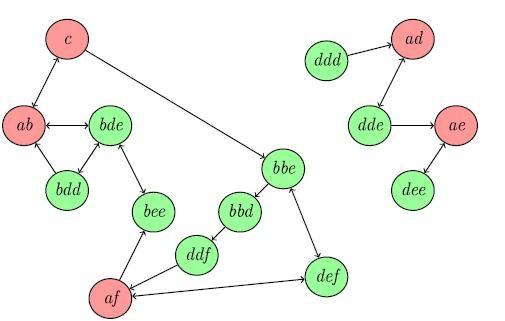Then the enumeration of all-pairs shortest paths in (X, Y) starting and ending in the elements of C = {c, ab, ad, ae, af} produces the following derivations:

\eqalign{ & c \to ab,\cr & c \to bbe \to def \to af,\cr & ab \to c,\cr & ab \to c \to bbe \to def \to af,\cr & ad \to dde \to ae,\cr & af \to bee \to bde \to ab,\cr & af \to bee \to bde \to ab \to c \cr}

.

Algorithm 3 extracts the set of all minimal acyclic metabolic pathways of length up to 2k, using the metabolites and reactions in R starting and ending in sets of at most m metabolites among those involved in the reactions in R, from the metabolic network (X, Y) produced by Algorithm 2.

In this algorithm, each path of the form u → …→ υ is extended in all possible ways by arcs in Y of the form υw until reaching an element wC, where the test wp ensures the resulting paths are acyclic.

Algorithm 3. Given a metabolic network (X, Y) and a set C of initial and final metabolite sets, enumerate all minimal acyclic metabolic pathways contained in (X, Y) which start and end in metabolite sets from C.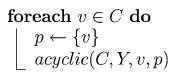where acyclic(C,E, υ, p) is defined as follows: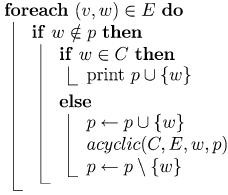Example 3. In the metabolic network (X, y) of Example 2, which corresponds to the toy artificial chemistry of Example 1, the enumeration of minimal acyclic paths starting and ending in the elements of C = {c, ab, ad, ae, af} produces the following derivations:

\eqalign{ & c \to ab,\cr & c \to bbe \to bbd \to ddf \to af\cr & c \to bbe \to def \to af,\cr & ab \to c,\cr & ad \to dde \to ae,\cr & af \to bee \to bde \to ab,\cr & af \to bee \to bde \to bdd \to ab \cr}

.

Remark 1. Notice that the shortest path derivation abcbbedefaf is not minimal, and the minimal acyclic derivation cbbebbdddfaf is not shortest.

## 4. Results and discussion

The size of an artificial chemistry is often exponential in the number of initial metabolites and biochemical reactions, and thus some method is needed for obtaining a significant portion of an artificial chemistry while avoiding the complexity of a complete reconstruction. The techniques we have introduced in this paper represent an important step in this direction, because they impose the only constraint on the reconstruction process that biochemical reactions be applied to combinations of at most m metabolites. Nevertheless, they allow for

1. (1)

Obtaining all pathways of length up to 2k by bidirectional search,

2. (2)

Storing them in a compact representation, and

3. (3)

Extracting shortest pathways and minimal acyclic pathways from the compact representation, where m and k are the only parameters of the reconstruction algorithms.

The metabolic reconstruction algorithm was implemented as a Perl script, using the Chemistry::Reaction module from the PerlMol collection of Perl modules for computational chemistry (Tubert-Brohman, 2004). The core of the methodology is embodied in the Chemistry::Artificial Perl module, which is available from the authors and will also be available from the PerlMol collection of Perl modules for computational chemistry (Tubert-Brohman, 2004). This module can be used to reconstruct the artificial chemistry defined by a given set of reaction equations written in reaction SMILES format (Weininger, 1988). For instance, the following Perl script first stores the artificial chemistry containing all derivations of length up to 2k = 4 starting and ending in sets of at most m = 2 metabolites using the reaction equations in file rctn.smi (Algorithm 2) and then, extracts all shortest derivations and all minimal acyclic derivations (Algorithm 3).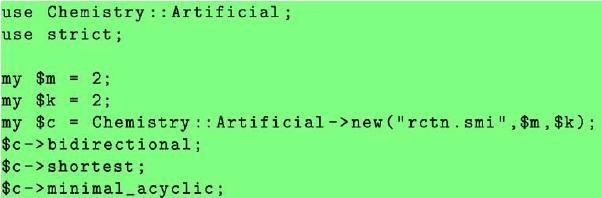We have performed a series of experiments in order to reconstruct metabolic pathways for all known reference pathway maps. The protocol we have used is as follows:

1. (1)

Obtain reference pathway maps from the KEGG (Kanehisa et al., 2006) database. We have used KEGG release 42.0 in all our experiments.

2. (2)

Solve the optimal atom mapping problem for all of the reactions in the reference pathways, using the optimal atom mapping by chemical substructure search algorithm and tool support (Félix and Valiente, 2007).

3. (3)

Reconstruct metabolic pathways of length up to 8 for each reference pathway.

4. (4)

Orient the reactions, according to the study of irreversibility of reactions in KEGG carried out in (Ma and Zeng, 2003).

5. (5)

Filter out those metabolic pathways that involve irreversible reactions applied in the reverse direction.

6. (6)

Identify the new metabolites thus obtained, by chemical structure search in CheBi (Brooksbank et al., 2005), MetaCyc (Caspi et al., 2006), KEGG (Kanehisa et al., 2006), and SciFinder Scholar (Wagner, 2006).

7. (7)

Analyze the new metabolic pathways for coexistence of metabolites and enzymes in each particular organism.

Preliminary results obtained by following the aforementioned experimental protocol upon 13 of the 308 reference pathway maps in KEGG are summarized in Tables 1 and 2. For the reference pathway map β-Alanine metabolism (00410), for instance, during the bidirectional chemical search for k = 1, the number of new metabolites was 264−106 = 158 and four new shortest pathways and also four new minimal acyclic metabolic pathways were obtained; for k = 2, the number of new metabolites was 293−158 = 135 and two new minimal acyclic metabolic pathways were obtained; and for k = 3, the number of new metabolites was 316−293 = 23, while no further new minimal acyclic pathway was found for k = 3, 4, and thus four new shortest pathways and six new minimal acyclic metabolic pathways were found while generating 7189 new metabolites.

The biological significance of these results can be assessed by examining the actual pathways found by bidirectional search, using the metabolites and reactions stored in KEGG for a particular reference pathway map. Besides obtaining again some of these reactions, an intermediate step is added in some metabolic pathways to one of the reactions stored in KEGG. For instance, using the metabolites and reactions stored in KEGG for glycine, serine, and threonine metabolism (reference pathway map 00260), we have obtained the following pathway: While the methylation of L-Serine to 2-Methylserine and demethylation of Pyruvate to Glyoxylate followed by the methylation of Glyoxylate to L-Alanine and demethylation of 2-Methylserine to Hydroxypyruvate is chemically feasible, the Serine pyruvate aminotransferase enzyme (2.6.1.51) allows for the oxidative deamination of L-Serine into L-Alanine, as stated in KEGG reaction R00585:

Among the novel metabolic pathways found by bidirectional search, using the metabolites and reactions stored in KEGG for carotenoid biosynthesis (reference pathway map 00906), we have obtained the following metabolic pathway: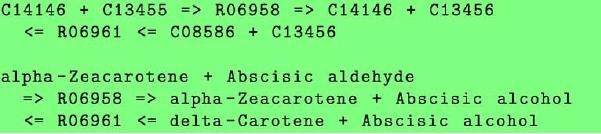A KEGG pathway reference map contains information for several organisms. Thus, it is important to find evidence that all four metabolites appearing in this pathway are present in a same organism, and also that the enzyme activating the reverse biochemical reaction R06961 (carotene 7,8-desaturase, 1.14.99.30) is indeed expressed in that particular organism.

Carotenoid biosynthesis spans several related pathways: spheroidene, normal-spirilloxanthin, unusual-spirilloxanthin, abscisic acid biosynthesis, and astaxanthin biosynthesis. However, there are organisms whose metabolism does not include both carotenoid biosynthesis and abscisic acid biosynthesis. In fact, Arabidopsis thaliana (thale cress) is the only organism for which the four metabolites are annotated in KEGG to carotenoid biosynthesis, and the gene coding for carotene 7,8-desaturase, AT3G04870, is indeed expressed in A. thaliana (Bartley et al., 1999; Scolnik and Bartley, 1995).

On the other hand, there is a biosynthetic pathway, the plastidic 2C-methyl-Derythritol 4-phosphate (MEP) pathway that involves the four metabolites and occurs in plastids, protozoa, most bacteria, and algae (Estévez et al., 2001). In the MEP pathway, carotenoid biosynthesis is a precursor of abscisic acid biosynthesis (Estévez et al., 2001, Fig. 1). In the novel metabolic pathway, alpha-Zeacarotene (C14146) and delta-Carotene (C08586) are involved in carotenoid biosynthesis whereas Abscisic aldehyde (C13455) and Abscisic alcohol (C13456) are involved in abscisic acid biosynthesis. Such a possible link between the early and later stages of the biosynthesis of steroids was established in (Estévez et al., 2001), where it is argued that only specific carotenoid intermediates (direct precursors of the abscisic acid biosynthesis) are increased or reduced, and further studied in (Seo and Koshiba, 2002) when regulating the early stages of abscisic acid biosynthesis in plants. The new metabolic pathway, shown in Fig. 2, is thus a novel pathway in the biosynthesis of carotenoid indeed.

While these preliminary results already reveal a number of new biochemical pathways, the artificial chemistry reconstruction starting from all sets of at most m metabolites among those involved in the set of reactions (the third constraint imposed on the reconstruction process) might reveal the existence of a much larger number of new biochemical pathways for m > 2. As can be seen in Table 3, the number of potential biochemical reactions grows fast with m for the reference maps stored in KEGG.

## References

• Bartley, G.E., Scolnik, P.A., Beyer, P., 1999. Two Arabidopsis thaliana carotene desaturases, phytoene desaturase and ζ-carotene desaturase, expressed in Escherichia coli, catalyze a poly-cis pathway to yield pro-lycopene. Eur. J. Biochem. 259(1–2), 396–03.

• Benkö, G., Flamm, C., Stadler, P.F., 2003a. Generic properties of chemical networks: artificial chemistry based on graph rewriting. In: Proc. 7th European Conf. Advances in Artificial Life, Lect. Notes Comput. Sci., vol. 2801, pp. 10–9. Springer, Berlin.

• Benkö, G., Flamm, C., Stadler, P.F., 2003b. A graph-based toy model of chemistry. J. Chem. Inf. Comput. Sci. 43(4), 1085–093.

• Benkö, G., Flamm, C., Stadler, P.F., 2004. Multi-phase artificial chemistry. In: Schaub, H., Detje, F., Brüggemann, U. (Eds.), The Logic of Artificial Life: Abstracting and Synthesizing the Principles of Living Systems, pp. 16–2. IOS Press, Amsterdam.

• Brooksbank, C., Cameron, G., Thornton, J., 2005. The European Bioinformatics Institute’s data resources: towards systems biology. Nucleic Acids Res. 33(D), D46–D53.

• Caspi, R., Foerster, H., Fulcher, C.A., Hopkinson, R., Ingraham, J., Kaipa, P., Krummenacker, M., Paley, S., Pick, J., Rhee, S.Y., Tissier, C., Zhang, P., Karp, P.D., 2006. MetaCyc: a multiorganism database of metabolic pathways and enzymes. Nucleic Acids Res. 34(D), D511–D516.

• Deville, Y., Gilbert, D., van Helden, J., Wodak, S.J., 2003. An overview of data models for the analysis of biochemical pathways. Brief. Bioinform. 4(3), 246–59.

• Dijkstra, E.W., 1959. A note on two problems in connexion with graphs. Numer. Math. 1(1), 269–71.

• Dittrich, P., Ziegler, J., Banzhaff, W., 2001. Artificial chemistries—a review. Artif. Life 7(1), 225–75.

• Edwards, J.S., Palsson, B.O., 2000. The Escherichia coli MG1655 in silico metabolic genotype: its definition, characteristics, and capabilities. P. Natl. Acad. Sci. USA 97(10), 5528–533.

• Estévez, J.M., Cantero, A., Reindl, A., Reichler, S., León, P., 2001. 1-deoxy-D-xylulose-5-phosphate synthase, a limiting enzyme for plastidic isoprenoid biosynthesis in plants. J. Biol. Chem. 276(25), 22901–2909.

• Félix, L., Rosselló, F., Valiente, G., 2007. Reconstructing metabolic pathways by bidirectional chemical search. In: Proc. 5th Int. Conf. Computational Methods in Systems Biology, Lect. Notes Bioinformatics, vol. 4695, pp. 217–32. Springer, Berlin.

• Félix, L., Valiente, G., 2007. Validation of metabolic pathway databases based on chemical substructure search. Biomol. Eng. 24(3), 327–35.

• Floyd, R.W., 1962. Algorithm 97: Shortest path. Commun. ACM 5(6), 345.

• Johnson, D.B., 1977. Efficient algorithms for shortest paths in sparse networks. J. ACM 24(1), 1–3.

• Kanehisa, M., Goto, S., 2000. KEGG: Kyoto encyclopedia of genes and genomes. Nucleic Acids Res. 28(1), 27–0.

• Kanehisa, M., Goto, S., Hattori, M., Aoki-Kinoshita, K.F., Itoh, M., Kawashima, S., Katayama, T., Araki, M., Hirakawa, M., 2006. From genomics to chemical genomics: New developments in KEGG. Nucleic Acids Res. 34(D), D354–D357.

• Karp, P.D., Mavrovouniotis, M.L., 1994. Representing, analyzing, and synthesizing biochemical pathways. IEEE Expert 9(2), 11–1.

• Lemer, C., Antezana, E., Couche, F., Fays, F., Santolaria, X., Janky, R., Deville, Y., Richelle, J., Wodak, S.J., 2004. The aMAZE LightBench: a web interface to a relational database of cellular processes. Nucleic Acids Res. 32(D), 443–48.

• Ma, H., Zeng, A.-P., 2003. Reconstruction of metabolic networks from genome data and analysis of their global structure for various organisms. Bioinformatics 19(2), 270–77.

• McCaskill, J., Niemann, U., 2001. Graph replacement chemistry for DNA processing. In: DNA 2000, Lect. Notes Comput. Sci., vol. 2054, pp. 103–16. Springer, Berlin.

• Michal, G. (Ed.), 1999. Biological Pathways: An Atlas of Biochemistry and Molecular Biology. Wiley, New York.

• Overbeek, R., Larsen, N., Pusch, G.D., D’Souza, M., Selkov, E., Kyrpides, N., Fonstein, M., Maltsev, N., Selkov, E., 2000. WIT: Integrated system for high-throughput genome sequence analysis and metabolic reconstruction. Nucleic Acids Res. 28(1), 123–25.

• Rosselló, F., Valiente, G., 2004. Analysis of metabolic pathways by graph transformation. In: Proc. 2nd Int. Conf. Graph Transformation, Lect. Notes Comput. Sci., vol. 3256, pp. 70–2. Springer, Berlin.

• Rosselló, F., Valiente, G., 2005a. Chemical graphs, chemical reaction graphs, and chemical graph transformation. Electron. Notes Theor. Comput. Sci. 127(1), 157–66.

• Rosselló, F., Valiente, G., 2005b. Graph transformation in molecular biology. In: Formal Methods in Software and System Modeling, Lect. Notes Comput. Sci., vol. 3393, pp. 116–33. Springer, Berlin.

• Schomburg, I., Chang, A., Schomburg, D., 2002. BRENDA, enzyme data and metabolic information. Nucleic Acids Res. 30(1), 47–9.

• Scolnik, P.A., Bartley, G.E., 1995. Nucleotide sequence of zeta-carotene desaturase (accession no. U38550) from arabidopsis. Plant Physiol. 109(4), 1499.

• Seo, M., Koshiba, T., 2002. Complex regulation of ABA biosynthesis in plants. Trends Plant Sci. 7(1), 41–8.

• Takaoka, T., 1998. Subcubic cost algorithms for the all pairs shortest path problem. Algorithmica 20(3), 309–18.

• Temkin, O.N., Zeigarnik, A.V., Bonchev, D., 1996. Chemical Reaction Networks: A Graph-Theoretical Approach. CRC Press, Boca Raton.

• Tubert-Brohman, I., 2004. Perl and chemistry. Perl J. 8(6), 3–5. PerlMol is available at http://www.perlmol.org/.

• Wagner, A.B., 2006. Scifinder scholar 2006: An empirical analysis of research topic query processing. J. Chem. Inf. Model. 46(2), 767–74.

• Weininger, D., 1988. SMILES, a chemical language and information system, 1: introduction to methodology and encoding rules. J. Chem. Inf. Comput. Sci. 28(1), 31–6. http://www.daylight.com/dayhtml/doc/theory/.

## Acknowledgement

The research described in this paper has been partially supported by the Spanish CICYT, project TIN 2004-07925-C03-01 GRAMMARS and project MTM2006-07773 COMGRIO, and by EU project INTAS IT 04-77-7178. A preliminary version of this paper has appeared in (Félix et al., 2007).

## Author information

Authors

### Corresponding author

Correspondence to Gabriel Valiente.

This work has been partially supported by the Spanish CICYT project TIN2004-07925-C03-01 GRAMMARS, by Spanish DGI projects MTM2006-07773 COMGRIO and MTM2006-15038-C02-01, and by EU project INTAS IT 04-77-7178.

## Rights and permissions

Open Access This is an open access article distributed under the terms of the Creative Commons Attribution Noncommercial License (https://creativecommons.org/licenses/by-nc/2.0), which permits any noncommercial use, distribution, and reproduction in any medium, provided the original author(s) and source are credited.

Reprints and Permissions

Félix, L., Rosselló, F. & Valiente, G. Efficient Reconstruction of Metabolic Pathways by Bidirectional Chemical Search. Bull. Math. Biol. 71, 750 (2009). https://doi.org/10.1007/s11538-008-9380-8